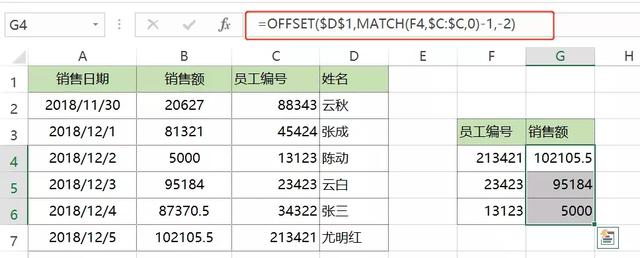# 财务函数，excel的常用函数公式大全

01

SUMPRODUCT函数

=SUMPRODUCT((条件1=条件区域1)*(条件2=条件区域2)*……*(求和区域))

=SUMPRODUCT((G5=B2:B9)*(H5=C2:C9)*D2:D9)，按Enter键完成。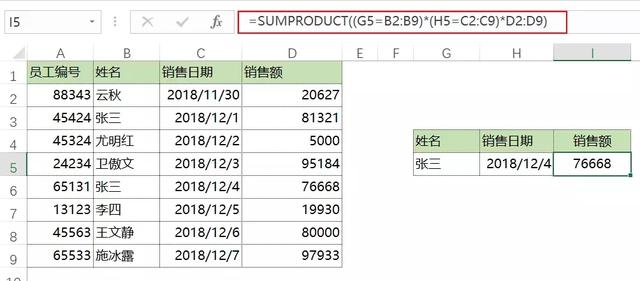02

LOOKUP函数

LOOKUP函数可以根据简称来查的全称。套路为：

=LOOKUP(1,0/(find(简称目标值,全称数据区域)),全称数据区域)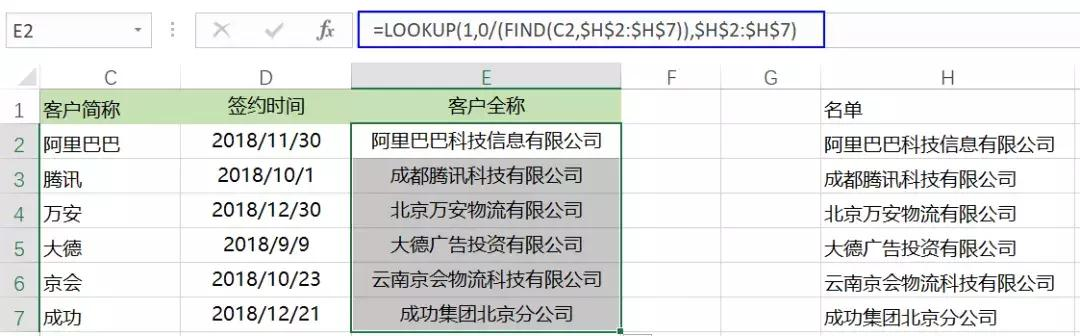03

IF函数

=if(条件表达式，条件成立时结果，不成立时结果)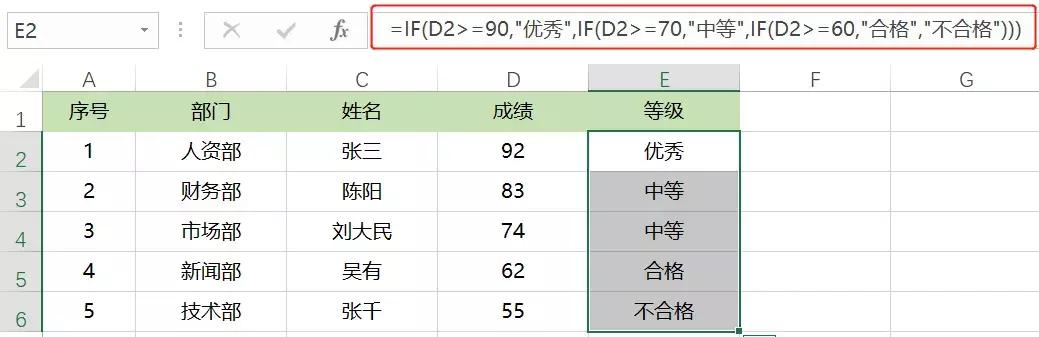=IF(D2>=90,”优秀”,IF(D2>=70,”中等”,IF(D2>=60,”合格”,”不合格”)))，然后确定向下填充。

04

AVERAGEIF函数

AVERAGEIF函数是多条件平均值函数。套路为：

=AVERAGEIF(求值区域，条件区域1，条件1，条件区域2，条件2……）

=AVERAGEIFS(G2:G7,A2:A7,”1月”,B2:B7,”1组”)，确定下拉即可。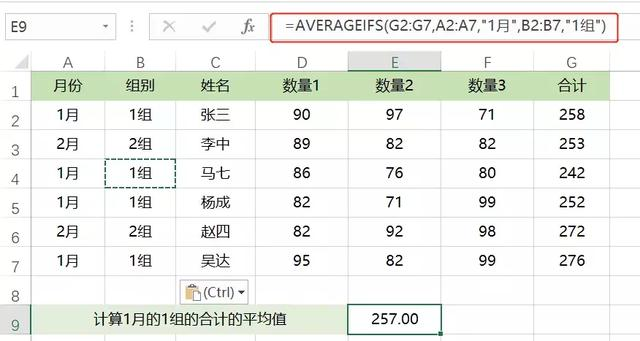05

DATEDIFIF函数

=DATEDIF(开始日期，结束日期，代码)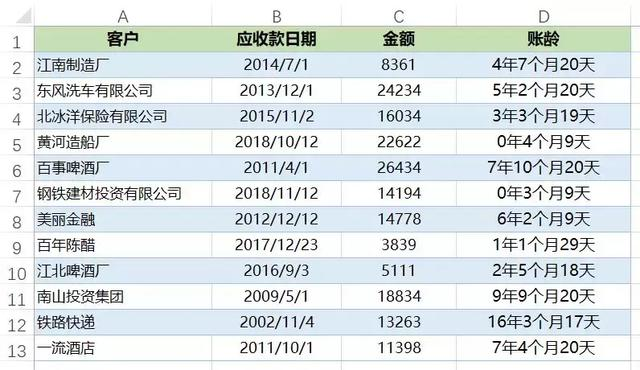=DATEDIF(B2,TODAY(),”y”)&”年”&DATEDIF(B2,TODAY(),”ym”)&”个月”&DATEDIF(B2,TODAY(),”md”)&”天”,按Enter键后完成向下填充即可。

06

VLOOKUP函数

VLOOKUP函数是最常用的函数，与MATCH函数配合可以发挥无穷的威力。套路为：

=VLOOKUP(查询，在那里查，第几列，精确/模糊查询）

=VLOOKUP(\$H3,\$A\$2:\$F\$13,MATCH(I\$1,\$A\$1:\$F\$1,0),0)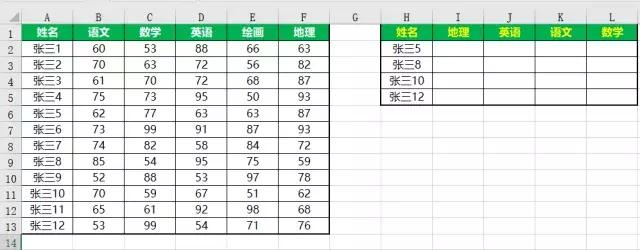07

WORKDAY函数

=WORKDAY(开始日期，结束日期，是否跳过节假日)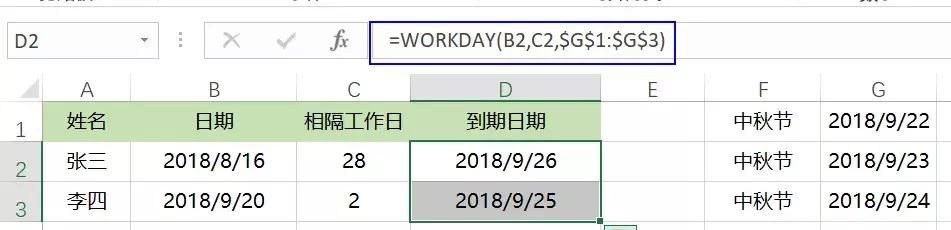08

OFFSET函数

=OFFSET(偏移对象，向下偏移的行数，向右偏移的列数，向下偏移高度，向右偏移宽度）